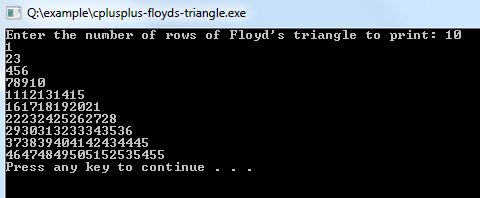## C++ Programming Examples Tutorial Index

A right-angled triangular array of natural numbers is called Floyd triangle.

Building a Floyd's triangle in C++ is quite easy, but you must have the understanding of how for loop works.

Example:

``````#include <iostream>
using namespace std;

int main()
{
int n, i,  c, a = 1;

cout << "Enter the number of rows of Floyd's triangle to print: "; cin >> n;

for (i = 1; i <= n; i++)
{
for (c = 1; c <= i; c++)
{
cout << a;
a++;
}
cout << endl;
}
return 0;
}
``````

Program Output:Explanation:

This program is used to print the right angled Floyd's triangle. For this you just need to understand the for-loop. So, first of all, you have to include the iostream header file using the "include" preceding by # which tells that the header file needs to be process before compilation, hence named preprocessor directive. Now, for removing naming conflict you can use namespace statement within a program.

Then the main() function is declared with return type as integer. Now you have to declare four integer type variables name as 'n', 'i', 'c' and 'a' and assign the value of 'a' as 1. Then the cout statement is used to display the message - "Enter the number of rows of Floyd's triangle to print: ". The cin statement eventually takes the value from the user and stores it in variable n.

Now, you have to use 2 for-loops in the form of nested for. First for loop will count using the variable i as 1and checks the condition i <= n. Again the second nested for-loop goes from c=1 till c <=i.

Then the cout statement is used within nested for to print all the numbers and increments the value of a by 1. After the inner loop gets executed, the cout takes the printing cursor to the next line.